# pylops.SecondDerivative#

class pylops.SecondDerivative(dims, axis=-1, sampling=1.0, kind='centered', edge=False, dtype='float64', name='S')[source]#

Second derivative.

Apply a second derivative using a three-point stencil finite-difference approximation along axis.

Parameters
dims

Number of samples for each dimension (None if only one dimension is available)

axisint, optional

New in version 2.0.0.

Axis along which derivative is applied.

samplingfloat, optional

Sampling step $$\Delta x$$.

kindstr, optional

Derivative kind (forward, centered, or backward).

edgebool, optional

Use shifted derivatives at edges (True) or ignore them (False). This is currently only available

for centered derivative

dtypestr, optional

Type of elements in input array.

namestr, optional

New in version 2.0.0.

Name of operator (to be used by pylops.utils.describe.describe)

Notes

The SecondDerivative operator applies a second derivative to any chosen direction of a multi-dimensional array.

For simplicity, given a one dimensional array, the second-order centered first derivative is:

$y[i] = (x[i+1] - 2x[i] + x[i-1]) / \Delta x^2$

while the second-order forward stencil is:

$y[i] = (x[i+2] - 2x[i+1] + x[i]) / \Delta x^2$

and the second-order backward stencil is:

$y[i] = (x[i] - 2x[i-1] + x[i-2]) / \Delta x^2$
Attributes
shapetuple

Operator shape

explicitbool

Operator contains a matrix that can be solved explicitly (True) or not (False)

Methods

 __init__(dims[, axis, sampling, kind, edge, ...]) adjoint() apply_columns(cols) Apply subset of columns of operator cond([uselobpcg]) Condition number of linear operator. conj() Complex conjugate operator div(y[, niter, densesolver]) Solve the linear problem $$\mathbf{y}=\mathbf{A}\mathbf{x}$$. dot(x) Matrix-matrix or matrix-vector multiplication. eigs([neigs, symmetric, niter, uselobpcg]) Most significant eigenvalues of linear operator. matmat(X) Matrix-matrix multiplication. matvec(x) Matrix-vector multiplication. reset_count() Reset counters rmatmat(X) Matrix-matrix multiplication. rmatvec(x) Adjoint matrix-vector multiplication. todense([backend]) Return dense matrix. toimag([forw, adj]) Imag operator toreal([forw, adj]) Real operator tosparse() Return sparse matrix. trace([neval, method, backend]) Trace of linear operator. transpose()

## Examples using pylops.SecondDerivative#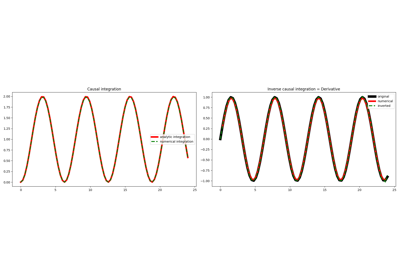Causal Integration

Causal Integration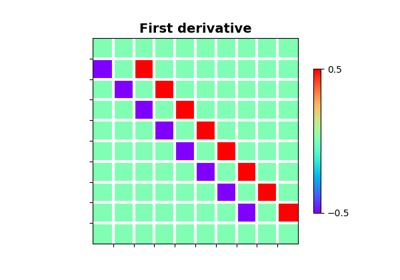Derivatives

Derivatives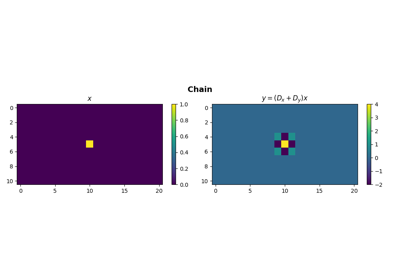Operators concatenation

Operators concatenation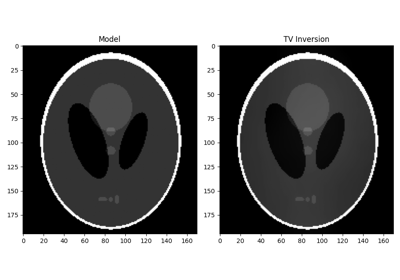Total Variation (TV) Regularization

Total Variation (TV) Regularization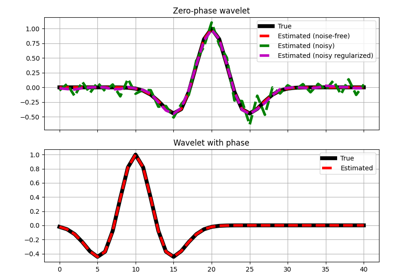Wavelet estimation

Wavelet estimation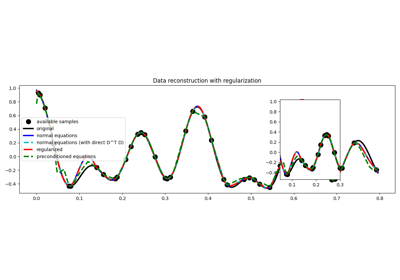03. Solvers

03. Solvers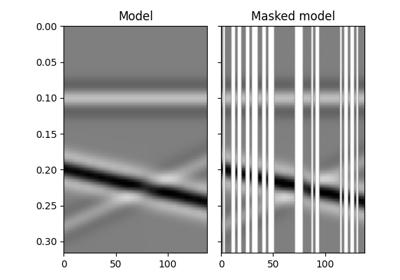12. Seismic regularization

12. Seismic regularization